New Students Offer - Use Code HELLO

# Alligation and Mixture : Tricks

Alligations is an important chapter for IBPS exams. This technique can be applied to many other chapters of Quantitative Aptitude. Today I will explain applications of Alligation method to various Quantitative Aptitude topics.
Ques 1. Gold is 19 times as heavy as water and copper 9 times. In what ratio should these materials be mixed so that the mixture may be 15 times as heavy as water.

Solution:-

Ques2.How much chicory at Rs. 4 a kg should be added to 15 kg. of teat Rs 10 a kg. so that the mixture be worth Rs. 6.50 a kg?
Solution:-

Ques3.A mixture of 40 litres of milk and water contains 10% water. How much water must be added to make water 20% in the new mixture?
Solution:-

Ques 4.A sum of Rs. 6.40 is made up of 80 coins which are either 10-paise or five-paise coins. How many are of 5-p.?
Solution:-

Ques 5.In a zoo, there are rabbits and pigeon. If heads are counted, there are 200 and if legs are counted, there are 580. How many pigeons are there?
Solution:-

Ques 6. A man has 30,000 rupees to lend on loan. He lent some of his capital to Mohan at an interest rate of 20% per annum and the rest to Suresh at an interest rate of 12% per annum. At the end of one year he got 17% of his capital as interest. How much did he lend to Mohan?
Solution:-
Ques 7. A vessel of 80 litre is filled with milk and water. 70% of milk and 30% of water is taken out of the vessel. It is found that the vessel is vacated by 53%. Find the initial quantity of milk and water
Solution:-
Ques 8.Mohan’s expenditure and savings are in the ratio os 4:1. His income increases by 20%. If his savings increase by 12%, by how much % should his expenditure increase?
Solution:-

Ques 9.Mukesh earned Rs. 4000 per month. From the last month his income increased by 8%. Due to rise in prices, his expenditure also increased by 12% and his savings decreased by 4%. Find his increased expenditure and initial saving.
Solution:-

Ques 10.A man has 60 pens. He sells some of these at a profit of 12% and the rest at 8% loss. On the whole, he gets a profit of 11%. How many pens were sold at 12% profit and how many 8% loss?
Solution:-

Qus11. The average weight of boys and girls is 25. The average weight of only boys is 30 and that of only girls is 15. If the no of boys is 24, find the no. of girls.
(A) 15
(B) 20
(C) 12
(D) 24
Sol:-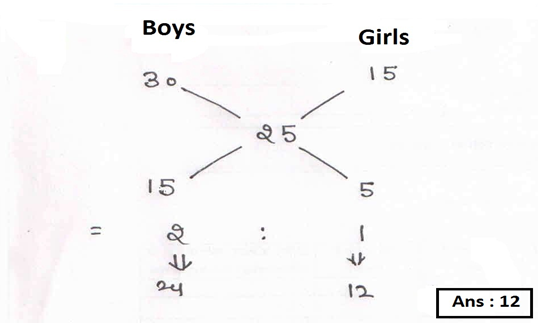Qus.12 17 liters of milk is mixed with 5 litre of water. If the price of water is 11per litre and that of milk is 33 per litre, find the average price.
(A) 30
(B) 32
(C) 26
(D) 28
Sol:-Qus.13 Khusbu travels at the speed of 25km/h for certain time. After this she travels at the speed of 40 km/h for 22 min. If the average speed of her journey is 31km/h find the time for which she travelled with the speed of 25km/h
(A) 30min
(B) 33 min
(C) 24 min
(D) 28 min
Sol:-Qus.14. Some amount of money was lent at 10% per annum and some at 20%per annum simple interest. Thus in 4 years, the total of interest earned from both the amount was rs 3400.If the total sum lent was 6000, find the amount lent at 20%.
(A) 3500
(B) 2500
(C) 2800
(D) 3000
Sol:-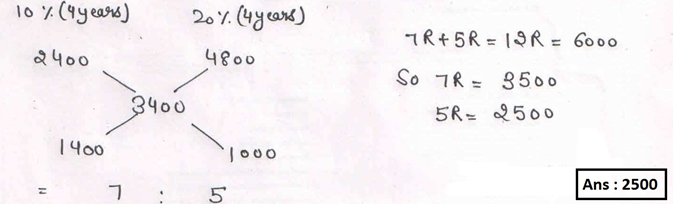Qus15. A sum of 41 was divided among 34 boys and 16 girls. The amount that each boy received was 90p then find the amount received by each girl.
(A) 65P
(B) 60P
(C) 62P
(D) 64P
Sol:-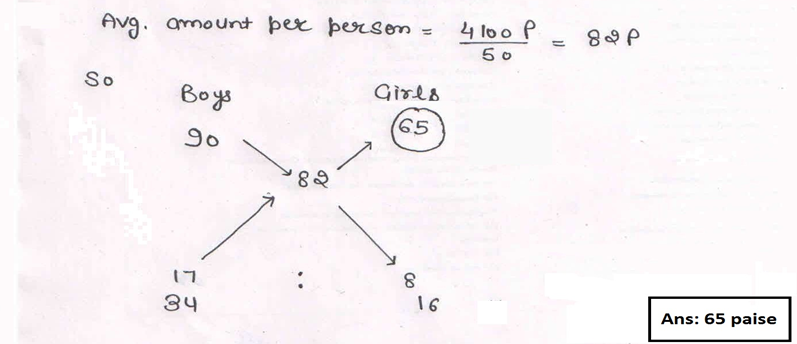Qus.16 Dharmender purchased a horse and a cow for Rs 150000. Then after some time he sold both of them. He sold the horse at a profit of 25% and the cow at a profit of 20%. But in the whole transaction he earned a total of Rs 33000 as profit. Find the cost price of horse.
(A) 90000
(B) 50000
(C) 60000
(D) 45000
Sol:-Qus.17 Vishal purchased 12 pencils and 4 pens at Rs240. He sold the pencils at of its cost price and the pens at of its cost price. If in the whole transaction he earned a profit of 2%, find the cost price of each pencil.
(A) 18
(B) 14
(C) 12
(D) 10
Sol:-Qus.18 35 liters of mixture of milk and water is sold Rs 60 per litre. The price of milk is 70 per litre and that of water is 20 per liter. Find the amount of milk in the mixture if the mixture is sold at a profit of 20%.
(A) 21L
(B) 35L
(C) 14L
(D) 26L
Sol:-Qus19. Two mixture of water and wine contain water and wine in the ratio of 7:3 and 1:4. In what ratio should these two mixtures should be mixed so as to obtain 40 liters of mixture is which the ratio of water and wine 3:2.
(A) 3:2
(B) 5:2
(C) 3:1
(D) 4:1
Sol:-Qus20. 21kg of alloy contains copper and tin in the ratio of 4:3. Some amount of copper is extracted so that the ratio becomes 10:9. Find the amount of copper extracted.
(A) 4kg
(B) 7kg
(C) 3kg
(D) 2kg
Sol:-Qus 21. In a mixture, the ratio of milk and water is 4:1. 75 liters of water is added to the mixture so that the ratio of milk and water becomes 2:3.Find the initial amount of milk in the mixture.
(A) 62L
(B) 60L
(C) 65L
(D) 75L
Sol:-Qus22. There are three vessels of equal capacity. The ratio of milk and water in these 3 vessels is 7:3, 7:5 & 11:4. Then the content of all these vessels are poured into a large vessel. Find the amount of milk and water in the resultant mixture.
(A) 121:95
(B) 112:59
(C) 121:59
(D) 25:12
Sol:-Qus23. There are two vessels containing wine and water in the ratio of 3:2 and 4:3. Mixture from both the vessels is taken out in the ratio of 2:3 and poured in a third large vessel. Find the amount of wine in the large vessel if the total amount of mixture in the large vessel is 350litres
(A) 175L
(B) 204L
(C) 200L
(D) 196L
Sol:-Qus.24. The ratio of oil and petrol in a container is 3:2. Then 10 litres of mixture is taken out and replaced by petrol so that after it the ratio of oil and petrol becomes 2:3. Find the initial amount of oil in the mixture.
(A) 9L
(B) 18L
(C) 20L
(D) 36L
Sol:-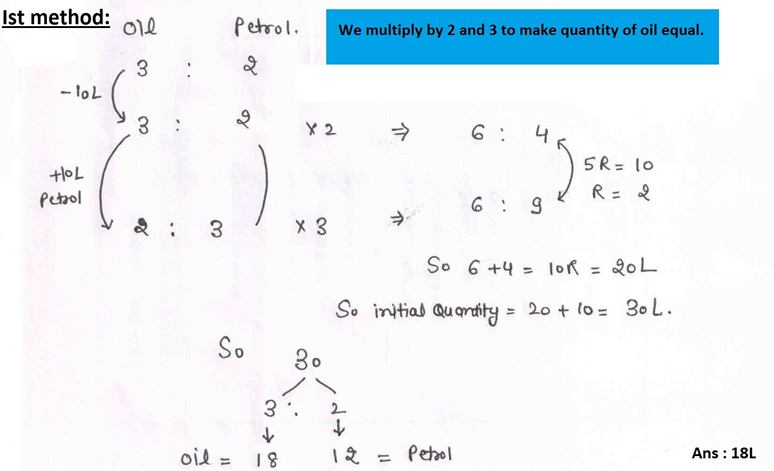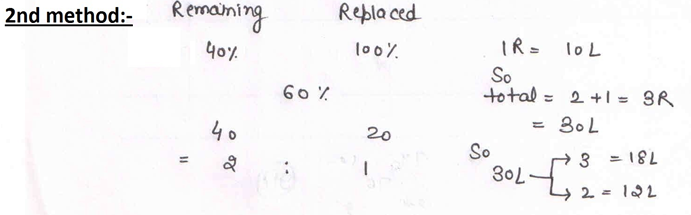Qus.25 Two vessels contain a mixture of milk and water. In the first vessel the ratio of milk to water is 8:3 and in the second vessel the ratio is 5:1. A 70 litres of cask is filled from these vessels so as the ratio of milk and water in the new cask is 4:1. Find the amount of mixture that was taken out from the first vessel.
(A) 14L
(B) 22L
(C) 20L
(D) 48L
Sol:-Qus.26 In a container the ratio of wine and soda is 4:1. 10 liters of mixture is drawn from it and replaced by 20 liters of soda. The final ratio of wine and soda becomes 2:3. Find the initial amount of soda in the mixture.
(A) 24L
(B) 20L
(C) 6L
(D) 10L
Sol:-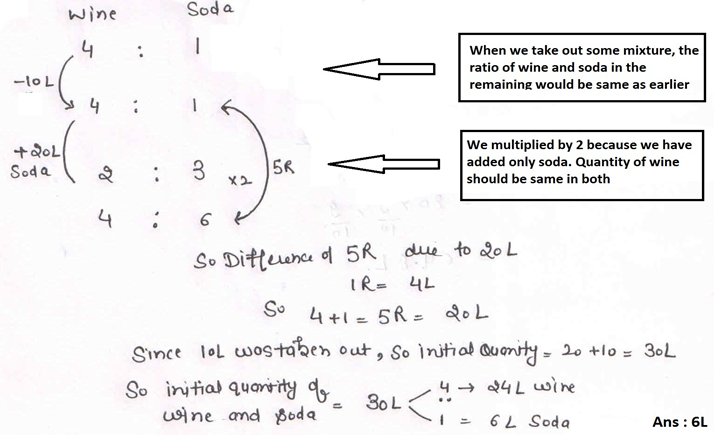Qus27. A bartender stole beer from a bottle that contained 50% of spirit and he replaced what he had stolen with beer having 20% spirit. The bottle then contained only 25% spirit. How much of the bottle did he steal.
(A) 6/11
(B) 5/11
(C) 6/5
(D) 5/6
Sol:-Qus.28 In a mixture of milk and water, the percentage of water is 26%. After replacing the mixture with 7 litres of pure milk, the percentage of milk in the mixture becomes 76%. Find the quantity of mixture.
(A) 84L
(B) 90L
(C) 91L
(D) 7L
Sol:-Qus29. A vessel is full of 80 litres of milk. 8 litres of milk is taken out and replaced by water. Again 8 litres of mixture is taken out and replaced by water, find the amount of milk in the final mixture so formed.
(A) 6.48
(B) 64.8
(C) 68
(D) 66.48
Sol:-Qus.30 9 litres is drawn from a cask full of water and then filled with milk. 9 litres of mixture is again drawn and replaced with milk. The quantity of water left in the cask to that of the milk is 16:9. How much does the cask hold.
(A) 45L
(B) 40L
(C) 144L
(D) 81L
Sol:-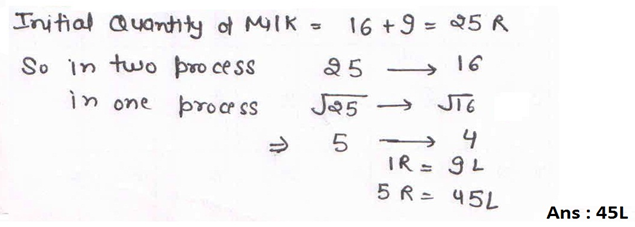Qus.31 From a container full of milk, 15 litres of milk was taken out and replaced by water. He again repeated the process for 2 more times. Thus in 3 attempts the ratio of milk to water becomes 343:169. Find the volume of container.
(A) 512L
(B) 120L
(C) 16L
(D) 214L
Sol:-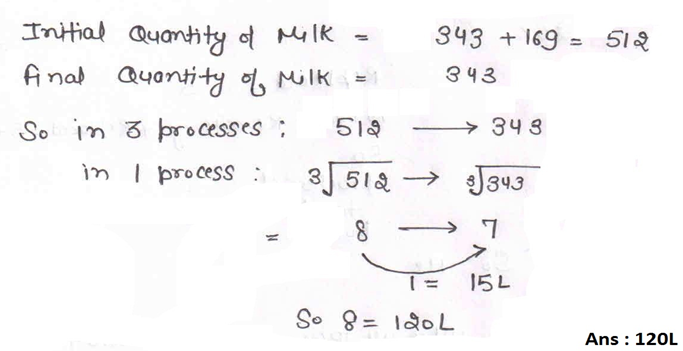Qus32. 2 vessels A & B of equal volume contain wine and soda in ratio of 3:2 and 2:1 respectively. 2 and 3 litres of solution is taken out from these vessles respectively and poured into a large vessel C. If this mixture occupied 40% of capacity of C, what proportion of volume of vessel C should be the volume of soda that shall be added so that the percentage of soda in vessel C becomes 50%.
(A) 144/160
(B) 14/144
(C) 14/125
(D) 12/127
Sol:-Qus33. A solution contain beer and soda in the ratio of 3:5. 20% of that solution is taken out and replaced by beer. How many times the process should be repeated to make the ratio of beer and soda 17:8.
(A) 2
(B) 3
(C) 4
(D) Can not be determined
Sol:-Qus 34. In a kerosene bottle there is 32% oil. Some quantity of kerosene is taken out and of that quantity is replaced by another kerosene that contain 18% oil so that the new mixture contain 28% oil. Find how much amount of kerosene is taken out .
(A) 2/7
(B) 3/4
(C) 4/9
(D) 6/11
Sol:-Qus 35. An mixture contains milk and water in the ratio of 2:3 by volume. In 15 liters of such mixture, 10 litres of milk is added then find what volume of water has to be removed from the mixture so that the final mixture has milk & water in the ratio of 4 : 1.
(A) 5.3L
(B) 5L
(C) 4.5L
(D) 5.67L
Sol:-